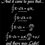# $\sqrt{-1}$ ?

If $\sqrt{-1}$ can be written as,

$\sqrt{-1} = \sqrt{1-2} = (1-2)^\dfrac{1}{2}$.

Now ,

$(1-2)^\dfrac{1}{2} = 1-\dfrac{1}{2}*(2)- \dfrac{1*1}{2*2*2}*(2^2)-\dfrac{1*1*3}{2*2*2*3!}*(2^3)-\cdots$

The above series will go upto infinite. So is this true? Is the value of $\sqrt{-1}$ is possible.Note by Akash Shukla
5 years, 1 month ago

This discussion board is a place to discuss our Daily Challenges and the math and science related to those challenges. Explanations are more than just a solution — they should explain the steps and thinking strategies that you used to obtain the solution. Comments should further the discussion of math and science.

When posting on Brilliant:

• Use the emojis to react to an explanation, whether you're congratulating a job well done , or just really confused .
• Ask specific questions about the challenge or the steps in somebody's explanation. Well-posed questions can add a lot to the discussion, but posting "I don't understand!" doesn't help anyone.
• Try to contribute something new to the discussion, whether it is an extension, generalization or other idea related to the challenge.

MarkdownAppears as
*italics* or _italics_ italics
**bold** or __bold__ bold
- bulleted- list
• bulleted
• list
1. numbered2. list
1. numbered
2. list
Note: you must add a full line of space before and after lists for them to show up correctly
paragraph 1paragraph 2

paragraph 1

paragraph 2

[example link](https://brilliant.org)example link
> This is a quote
This is a quote
    # I indented these lines
# 4 spaces, and now they show
# up as a code block.

print "hello world"
# I indented these lines
# 4 spaces, and now they show
# up as a code block.

print "hello world"
MathAppears as
Remember to wrap math in $$ ... $$ or $ ... $ to ensure proper formatting.
2 \times 3 $2 \times 3$
2^{34} $2^{34}$
a_{i-1} $a_{i-1}$
\frac{2}{3} $\frac{2}{3}$
\sqrt{2} $\sqrt{2}$
\sum_{i=1}^3 $\sum_{i=1}^3$
\sin \theta $\sin \theta$
\boxed{123} $\boxed{123}$

Sort by:

The convergence of the binomial expansion

$(1+x)^n = 1+ nx + \dfrac{n(n-1)}{2!} x^2 + \dfrac{n(n-1)(n-2)}{3!} x^3 + \cdots$

is only true for $-1\leq x<1$.

- 5 years, 1 month ago

Thank you very much. Now I got this. But the above series is divergence , then can we apply this?

- 5 years, 1 month ago

No. The binomial theorem is valid only when the power is an integer.

- 5 years, 1 month ago

Then what we use for expansion $\sqrt{x+1}$ or such others quantities.

- 5 years, 1 month ago